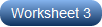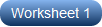# Multiplying Fractions on a Number Line Worksheets

Multiplying fractions on number lines worksheet compilation is your ticket to a great start. The number line model is an arithmetic strategy that helps kids immensely in carrying out operations on fractions. The number line is divided into equal segments and calibrated in fractions. The number of hops is the multiplicand, a whole number in this case; the point where the first hop ends is the multiplier, and the point where the last hop ends is the product. Give kids a vivid picture of the parts of the number line as a prelude to the exercises that follow. Commence practice with drawing hops on the number line model to find the product, proceed to writing the multiplication sentence by observing the model, and wrap up with writing the missing part in the multiplication equation. With this wide variety of printable practice worksheets, we leave no stone unturned for your 4th grade and 5th grade kids. Our free multiplying fractions on number lines worksheet with an answer key is an ideal starter.

Multiplying Fractions by Whole Numbers

Make kids adept in finding the product of a fraction and a whole number to do simple everyday calculations as they walk through these pdfs. Get kids to draw hops on the number line based on the equation. The point where the last hop ends is your product.Writing Multiplication Equations from Number Lines

Detect the whole number multiplicand, the multiplier, and the product from the number line. Put these together to frame a multiplication equation. Writing the fraction multiplication sentence is no hard row to hoe with the number line models.Write the Missing Fractions

Whether it is the multiplicand, the multiplier, or the product that is missing in the equations featured in this unit of fraction multiplication on number lines worksheets, kids in 4th grade and 5th grade figure it out and plug it in the multiplication sentences.